## 5.1.4 Deposition Models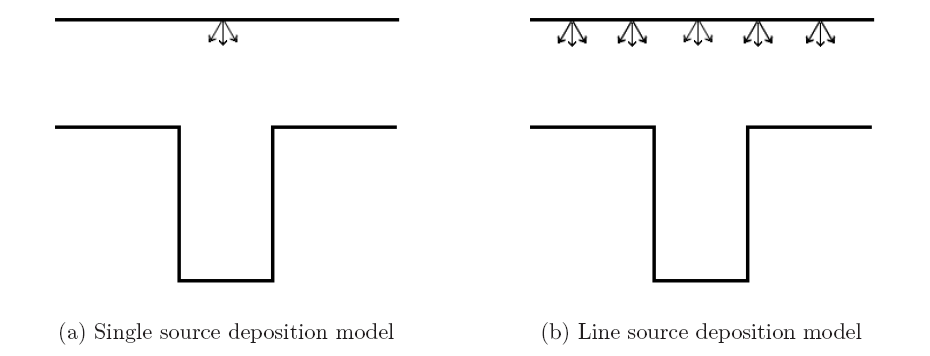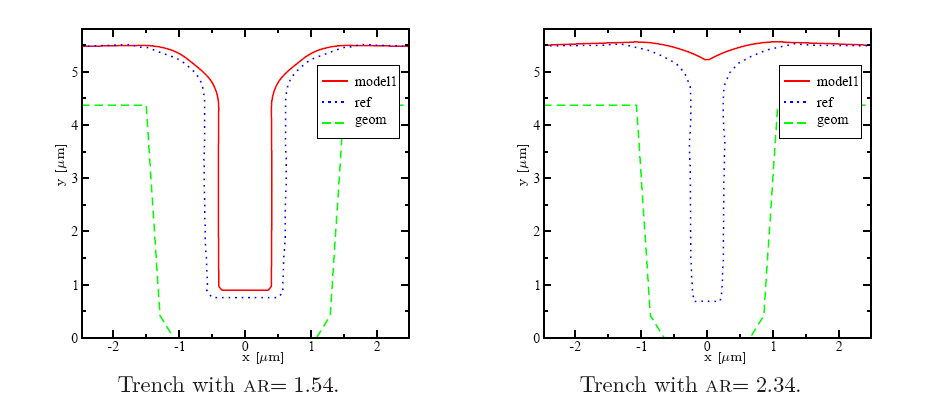In this section, the most promising models are described together with the best parameter sets which lead to the most accurate simulation results for the TEOS deposition in a certain AR range. The simulations have been performed using the topography simulator ELSA (Enhanced Level Set Applications) which follows the surface evolution by solving the level set equation [319,333]. The parameter calibration and the optimizations have been carried out using the simulation and optimization framework SIESTA (cf.  and Section 4.5).

In an early attempt a model based on a single point-shaped material source was considered . SIESTA was used to identify the sticking coefficients of the model and to tune the model, the distance between the source and the upper trench surface. The optimization has been performed for a trench with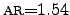. In Figure 5.3a a calibration result for this particular trench is presented. However, if this model is applied to trenches with a higher AR, the simulation results become worse and do neither quantitatively nor qualitatively compare with the measurement for an increased AR above approximately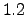as is presented in Figure 5.3b for trenches with AR of 1.54 and 2.34. Here, the latter trench is completely closed in contrast to the measurements. Nevertheless, for planar surfaces as well as for trenches with very low aspect ratios, this model gives reasonable good results.

Since the previous approach delivers only satisfactory results for a very limited set of trenches, the single source model has been expanded to a continuous line-source model where the material flux depends only on the visible angle between the surface elements and the source. The model describes the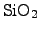deposition by two sticking coefficients, where the first sticking coefficient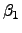describes the sticking probability of the incoming TEOS particles. As already mentioned, when the TEOS molecule hits the hot wafer surface, the TEOS molecule is pyrolytically dissociated. The probability that thewill stick at the surface is modeled with the constant sticking coefficient. With a certain probability (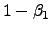), the particle is reflected from the first contact and hits the surface a second time. The probability that theis deposited at the second surface hit is described with the second sticking coefficient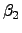.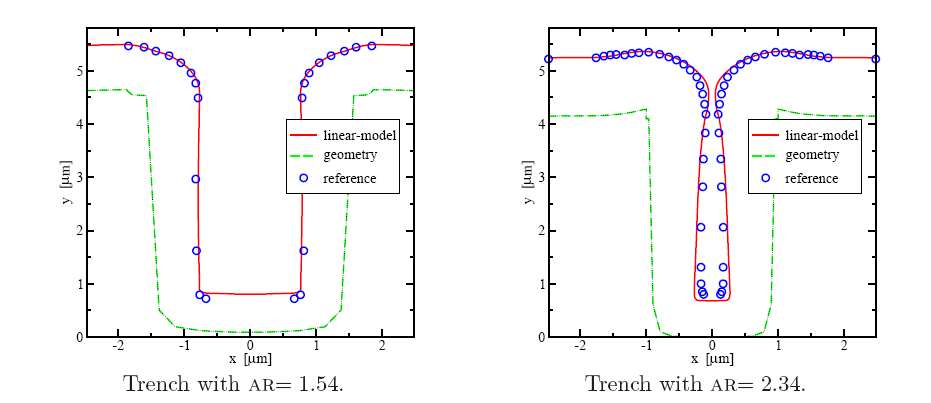Compared to the earlier attempt, the problem that the top trench closes withat larger ARs, is shifted to higher ARs compared to the previous model, but the geometry at the bottom of the trench does still not satisfactorily agree with measurement as depicted in Figure 5.4. The corresponding parameters were calibrated with SIESTA, where the coefficients for the sticking probabilityandwere identified as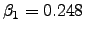and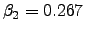. Another improvement has been achieved by using a single flux-dependent sticking model . The deposition reaction follows a half order kinetics of a CVD process of TEOS. Hence, the sticking coefficient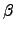is modeled to be proportional to the inverse of the square root of the local mass flux coming from the source as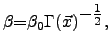(5.4)

where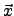is the local position,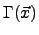the local material flux, and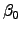a constant scaling factor for calibration purposes. For the current LPCVD TEOS deposition process, the scaling factorwas identified as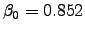with good agreement to the measurements for low aspect ratios as shown in Figure 5.5a. However, trenches with higher aspect ratios (cf. Figure 5.5b) show a considerable overestimation of the material thickness on the side walls of the trenches may result in spurious void formations for arbitrarily shaped trenches.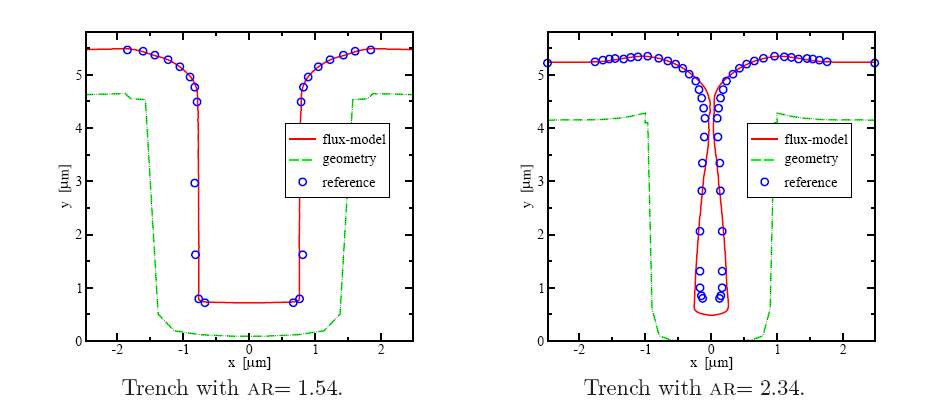With increasing AR the overestimation of the thickness at the bottom increases as shown in Figure 5.4b and Figure 5.5b. Thus, another deposition model has been developed which considers two statistically independent species taking part in the TEOS deposition reaction. The first species is the original TEOS which is dissociated at the wafer surface. The second species is a sum of all other by-products of this pyrolytic reaction for which an average sticking probability is assigned. Since this model considers as initial condition a uniform distribution of reactants in the reactor, the sticking probability for both types of species can be assumed to be statistically independent. However, there is a dependence that the mass flux of the chemical by-products has to be proportional to the incoming material flux of TEOS due to mass conservation. With the assumption that the TEOS deposition is a LPCVD process, the TEOS particles are in a rather low concentration in the reactor and have a large mean free path between collisions. Thus, the material source for the reactor has to be sufficiently provide TEOS inside the reactor to obtain a stable deposition process. Since this requires a high exchange rate of the gases inside the reactor according to balance the high consumption rate of TEOS, the ratio between the TEOS concentration and the concentration of the by-products can be assumed to be in average constant. Despite of this fact the second species is modeled with an additional constraint which guarantees that the flux of the second species is proportional to the reflections of the first species because the second species is a product from the dissociation of the TEOS particles. If, for instance, trenches with higher ARs are considered, the material deposition at the bottom of the trench is much less than at the top because the distance form the TEOS source to the bottom of the trench is much wider and the by-products cannot be exhausted as quickly as from the top side of the trench. Hence, the flux of the second species is modeled to be proportional to the flux of the first species but with a statistically independent sticking probability.

This new two-species model shows excellent agreement with measurements for different geometries as shown in Figure 5.6a and Figure 5.6b. The sticking coefficientandwere identified with SIESTA as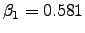and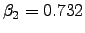. In addition to the astonishing agreement, this model provides faster simulation results and requires only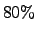of the CPU time compared to the flux-dependent deposition model and overcomes the overestimation at the side walls of the trenches. Thus, the presented method enables efficient and accurate geometry optimizations. The hereby extracted sticking coefficients have also been applied to three-dimensional structures and have shown promising results.Stefan Holzer 2007-11-19